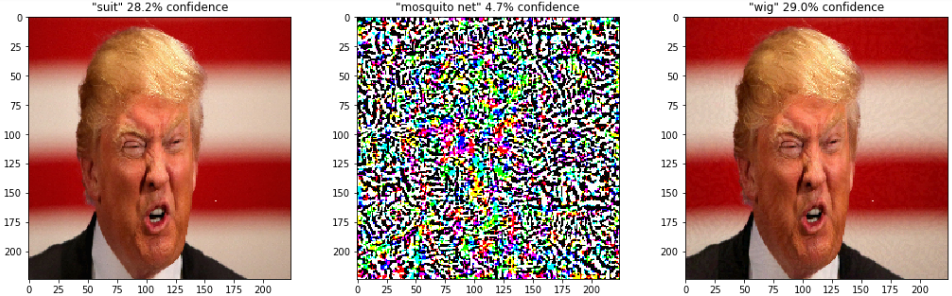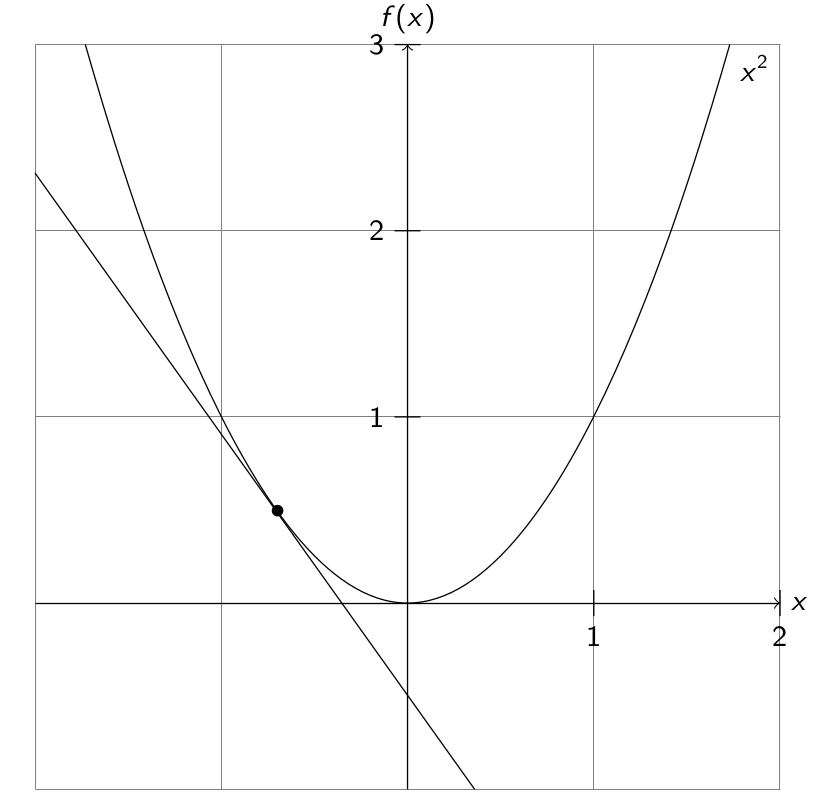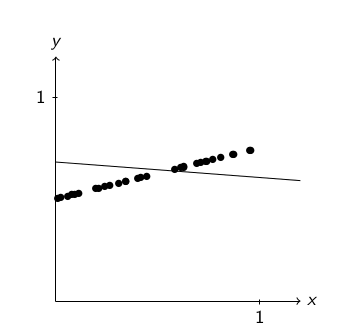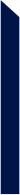Search
• GREGORY CHATEL

# Adversarial examples in deep learning

Updated: Nov 13, 2019

This post will contain essentially the same information as the talk I gave during the last Deep Learning Paris Meetup. I feel that as more and more fields start to use deep learning in critical systems, it is important to bring awareness on how neural networks can be fooled to produce strange and potentially dangerous behaviors. The goal of this post is not to be as mathematically precise and exhaustive as possible but to give you a basic understanding of adversarial examples and hopefully to sensibilize you to one of the problems of modern day deep learning.

So first, what are adversarial examples?

An adversarial example is a sample of input data which has been modified very slightly in a way that is intended to cause a machine learning to misclassify it.

An example should help anchor the idea, let's compute an adversarial example from a Donald Trump image.An image classified as “suit” by the VGG16 neural network (left), a perturbation created specifically using the image on the left (middle) and the resulting perturbed image classified as a wig (right).

In this figure, we can see that the classification of the perturbed image (rightmost) is clearly absurd. We can also notice that the difference between the original and modified images is very slight.

With article like California’s finally ready for truly driverless cars or The Pentagon’s ‘Terminator Conundrum’: Robots That Could Kill on Their Own, applications of adversarial examples are not exactly hard to come up with…

In this post I will first explain how such images are created and then go through the main defenses that have been published. For more details and more rigorous information, please refer to the research papers referenced.

First, a quick reminder on gradient descent. Gradient descent is an optimization algorithm used to find a local minimum of a differentiable function.A curve and a tangent to this curve.

In the figure on the left, you can see a simple curve. Suppose that we want to find a local minimum (a value of x for which f(x) is locally minimal).

The gradient descent consists in the following steps: first pick an initial value for x, then compute the derivative f’ of f according to x and evaluate it for our initial guess. f’(x) is the slope of the tangent to the curve at x. According to the sign of this slope, we know whether we have to increase or decrease x to make f(x) decrease. In the example on the left, the slope is negative so we should increase the value of x to make f(x) decrease. As the tangent is a good approximation of the curve in a tiny neighborhood, the value change applied to x is very small to ensure that we do not jump too far.Suppose that we have a set of points and we want to find a line that is a reasonable approximation for these values.

Our machine learning model will be the line y = ax + b and the model parameters will be a and b.

Now to use the gradient descent, we are going to define a function of which we will want to find a local minimum. This is our loss function.This loss takes a data point x, its corresponding value y and the model parameters a and b. The loss is the squared difference of the real value y and ax + b, the prediction of our model. The bigger the difference between the real and predicted values is, the bigger the value of the loss function will be. Intuitively, we chose the squaring operation to penalize big differences between real and predicted values more than small ones.

Now we compute the derivative of our function L according to the parameters of the model a and b.And as before, we can evaluate this derivatives with our current values of a and b for each data point (x, y), which will give us the slopes of the tangents to the loss function and use these slopes to update a and b in order to minimize L.

OK, that’s cool and all but that’s not how we’re going to generate our adversarial examples…

Well, in fact it is exactly how we are going to do it. Suppose now that the model is fixed (you can’t change a and b) and you want to increase the value of the loss. The only thing left to modify are the data points (x, y). As modifying the ys does not really make sense, we will modify the xs.

We could just replace the x by random values and the loss value would increase by a tremendous amount but that’s not really subtle, in particular, it would be really obvious to a human plotting the data points. To make our changes in a way that is not obviously detected by an observer, we will compute the derivative of the loss function according to x.And now, just as before, we can evaluate this derivative on our data points, get the slope of the tangent and update the x values by a small amount accordingly. The loss will increase and, as we are modifying all the points by a small amount, our perturbation will be hard to detect.

Well that was a very simple model that we’ve just messed with, deep learning is much more complicated than that…

Guess what? It’s not. Everything we just did has a direct equivalent in the world of deep learning. When we are training a neural network to classify images, the loss function is usually a categorical cross entropy, the model parameters are the weights of the network and the inputs are the pixel values of the image.

The basic algorithm of adversarial sample generation, called Fast Gradient Sign Method (from this paper), is exactly what I described above. Let’s explain it and run it on an example.

Let x be the original image, y the class of x, θ the weights of the network and L(θ, x, y) the loss function used to train the network.First, we compute the gradient of the loss function according to the input pixels. The operator is just a concise mathematical way of taking the derivatives of a function according to many of its parameters. You can think of as a matrix of shape [width, height, channels] containing the slopes of the tangents.As before, we are only interested in the sign of the slopes to know if we want to increase or decrease the pixel values. We multiply these signs by a very small value ε to ensure that we do not go too far on the loss function surface and that the perturbation will be imperceptible. This will be our perturbation.Our final image is just our original image to which we add the perturbation η.

Let’s run it on an example:FGSM applied to an image. The original is classified as ‘king penguin’ with 100% confidence and the perturbed one is classified as ‘tripod’ with 71% confidence.

The family of attack where you are able to use compute gradients using the target model are called white-box attacks.

Now you could tell me that the attack I’ve just presented is not really realistic as you’re unlikely to get access to the gradients of the loss function on a self-driving car. Researchers thought exactly the same thing and, in this paper, they found a way to deal with it.

In a more realistic context, you would want to attack a system having only access to its outputs. The problem with this is that you would not be able to apply the FGSM algorithm anymore as you would not have access to the network itself.

The solution proposed is to train a new neural network M’ to solve the same classification task as the target model M. Then, when M’ is trained, use it to generate adversarial samples using FGSM (which we now can do since it is our own network) and ask M to classify them.

What they found is that M will very often misclassify adversarial samples generated using M’. Moreover, if we do not have access to a proper training set for M’, we can build one using M predictions as truth values. The authors call this synthetic inputs. This is an excerpt of their article in which they describe their attack on the MetaMind network to which they did not have access:

“After labeling 6,400 synthetic inputs to train our substitute (an order of magnitude smaller than the training set used by MetaMind) we find that their DNN misclassifies adversarial examples crafted with our substitute at a rate of 84.24%”.

This kind of attack is called black-box attack as you see the target model as a black-box.

So, even when the attacker does not have access to the internals of the model he can still produce adversarial sample that will fool it but still, this attack context is not realistic either. In a real scenario, the attacker would not be allowed to provide its own image files, the neural network would take camera pictures as input. That’s the problem the authors of this article are trying to solve.

What they noticed is that when you print adversarial samples which have been generated with a high-enough ε and then take a picture of the print and classify it, the neural network is still fooled a significant portion of the time. The authors recorded a video to showcase their results:

“We used images taken from a cell-phone camera as a input to an Inception v3 image classification neural network. We showed that in such a set-up, a significant fraction of adversarial images crafted using the original network are misclassified even when fed to the classifier through the camera.”

Now that the potential for an attack in a realistic context seems reasonable, let’s review a few defense strategies.

The first idea that comes to mind when trying to defend adversarial examples is usually to generate a lot of them and run more training passes on the network with these images and the correct class as targets. This strategy is called adversarial training.

While this strategy improves robustness to FGSM attacks, it has multiple downside:

• It does not help against more sophisticated white-box attacks likeRAND+FGSM, which, as explained in the article, we cannot use to adversarially train a network.

• It does not help against black-box attacks either.

This last point is quite surprising but true and have been observed in multiple contexts. The reasons of this behavior are explored in this paper. Proper white-box defense is something that we do not know how to do perfectly as I’m writing this post. As the authors note, the fact that adversarially trained networks are still vulnerable to black-box attacks is often not taken into account in articles that presents new defense strategies.

While white-box defense seems like a difficult problem, the hypothesis that the attacker has access to the model weights is big. Researchers tried to produce networks that are robust to black-box attacks in this paper.

Since adversarially trained networks are still vulnerable to black-box attacks, the authors propose Ensemble Adversarial Training, a strategy which consists in adversarially training a model using modified samples from an ensemble of other models (usually 2 to 5). For example, they train a model to classify MNIST digits using adversarial examples crafted using 5 other pre-trained models. The error rate goes from 15.5% for an adversarially trained model to 3.9% for an ensemble adversarially trained model for a black-box attack.

This algorithm is the best so far at black-box defense.

As a conclusion on the defense part, I will cite a blog post from I. Goodfellow and N. Papernot:

“Most defenses against adversarial examples that have been proposed so far just do not work very well at all, but the ones that do work are not adaptive. This means it is like they are playing a game of whack-a-mole: they close some vulnerabilities, but leave others open.”

If you enjoyed reading this blog post and would like to know if and how your company's models are impacted by this kind of vulnerabilities, don't hesitate to contact us.